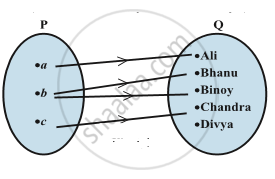# Relation

#### description

• Definition of Relation
• Domain
• Co-domain and Range of a Relation

#### definition

A relation R from a non-empty set A to a non-empty set B is a subset of the cartesian product  A × B. The subset is derived by describing a relationship between the first element and the second element of the ordered pairs in A × B. The second element is called the image of  the first element.

The set of all first elements of the ordered pairs in a relation R from a set A to a set B is called the domain of the relation R.

The set of all second elements in a relation R from a set A to a set B is called the range of the relation R. The whole set B is called the codomain of the relation R. Note that range ⊂ codomain.

#### notes

(i) A relation may be represented algebraically either by the Roster method or by the Set-builder method.
(ii) An arrow diagram is a visual representation of a relation.
Consider the two sets P = {a, b, c} and Q = {Ali, Bhanu, Binoy, Chandra, Divya}.The cartesian product of P and Q has 15 ordered pairs which can be listed as P × Q = {(a, Ali), (a,Bhanu), (a, Binoy), ..., (c, Divya)}. We can now obtain a subset of P × Q by introducing a relation R between the first element x and the second element y of each ordered pair (x, y) as R= { (x,y): x is the first letter of the name y, x ∈ P, y ∈ Q}. Then R = {(a, Ali), (b, Bhanu), (b, Binoy), (c, Chandra)} A visual representation of this relation R (called an arrow diagram) is shown in Fig .
A relation R from A to A is also stated as a relation on A.

If you would like to contribute notes or other learning material, please submit them using the button below.

### Shaalaa.com

RELATIONS [00:12:40]
S
0%